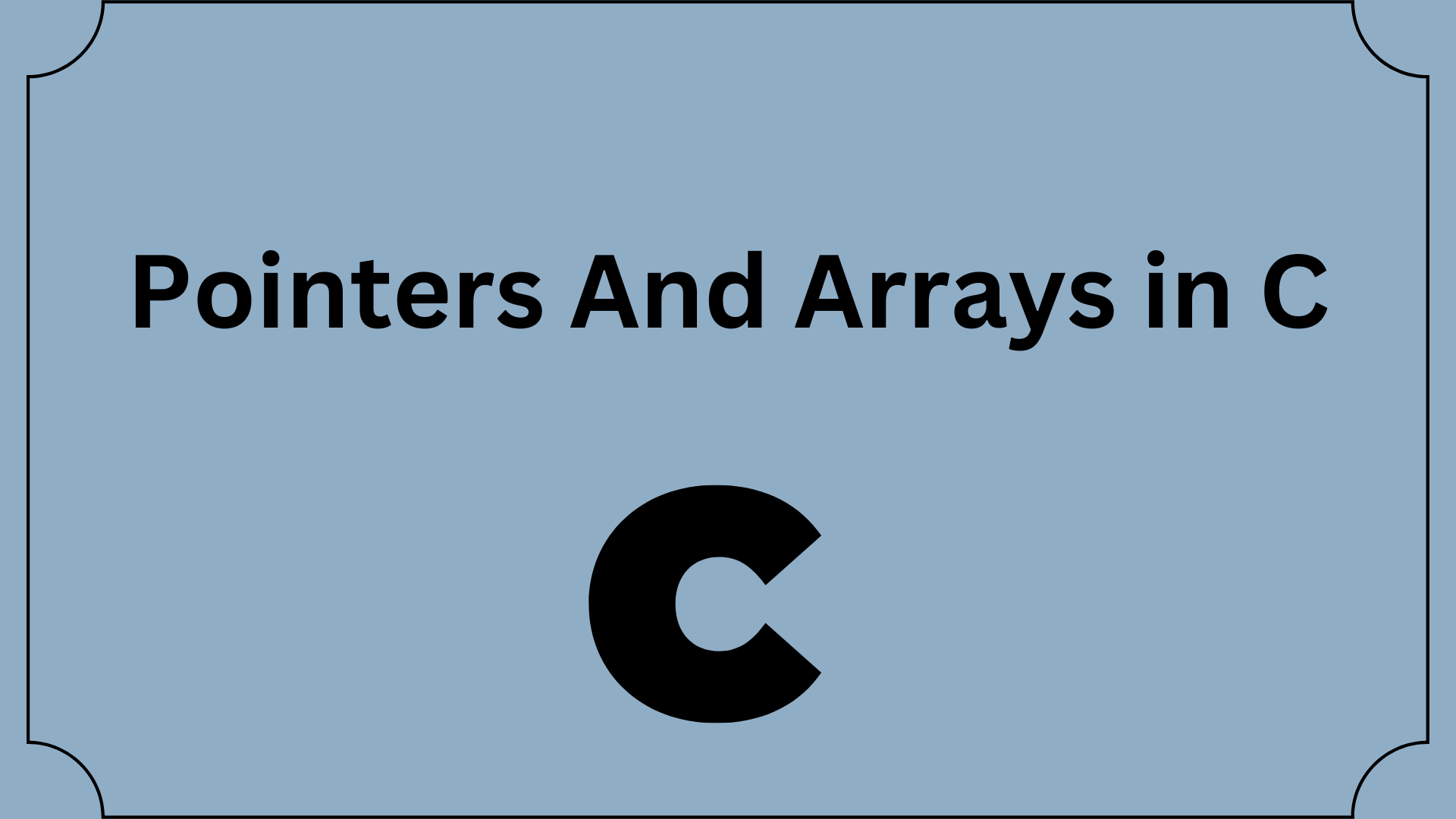## Pointers And Arrays In C Programming Language

In this tutorial, you’ll learn about the relationship between Pointers And Arrays In C programming. You will also learn to access array elements using pointers.

An array variable is similar to a pointer that always points to the first element of the array in terms of memory address; it cannot be incremented like a pointer variable, but it may be used to point to different array elements in arithmetic expressions.

You discovered that a pointer to a specific data type can store the address of any variable of that specific data type in a previous tutorial on pointers. The address of the character variable c is stored in the pointer variable pc, for instance, in the code below.

```char c = 'A';
char *pc = &c;```

In this case, the variable c is a scalar, meaning it can only have one value. The arrays that can store many instances of the same data type in a single, continuously allocated memory block are already familiar to you. You might then ask, “Can we also have pointers to arrays?” We can, in fact.

Before you learn about the relationship between arrays and pointers, be sure to check these two topics:

• C Arrays
• C Pointers

Contents

## Relationship Between Pointers And Arrays In C

A block of consecutive data is called an array. Let’s create a program that prints array element addresses.

```#include <stdio.h>
int main() {
int x;
int i;

for(i = 0; i < 4; ++i) {
printf("&x[%d] = %p\n", i, &x[i]);
}

printf("Address of array x: %p", x);

return 0;
}```

## Output

&x = 1450734448
&x = 1450734452
&x = 1450734456
&x = 1450734460

The distance between any two consecutive array x elements is 4 bytes. It is a result of int’s 4-byte size (on our compiler).

Keep in mind that x and &x have the same address. It is thus because the variable x refers to the array’s initial element.

It is evident from the example above that &x is equivalent to x. Moreover, *x is identical to x.

Similarly,

• Both &x and x are identical to x+1 and *(x+1), respectively.
• Both &x and x are equivalent to x+2 and *(x+2), respectively.
• In essence, &x[i] and x[i] are equal to x+i and *(x+i), respectively.

## Example 1: Pointers and Arrays

```#include <stdio.h>
int main() {

int i, x, sum = 0;

printf("Enter 6 numbers: ");

for(i = 0; i < 6; ++i) {
// Equivalent to scanf("%d", &x[i]);
scanf("%d", x+i);

// Equivalent to sum += x[i]
sum += *(x+i);
}

printf("Sum = %d", sum);

return 0;
}```

The following will appear after you execute the program:

Enter 6 numbers: 2
3
4
4
12
4
Sum = 29

Here, an array x with six elements has been declared. We have utilized pointers to access the array’s elements.

The majority of the time, array names become pointers. In plain English, array names are changed into pointers. You can access array elements via pointers because of this. But keep in mind that pointers and arrays are not the same things.

Array names occasionally do not degrade to pointers. Visit When does array name doesn’t decay into a pointer for more information.

## Example 2: Arrays and Pointers

```#include <stdio.h>
int main() {

int x = {1, 2, 3, 4, 5};
int* ptr;

// ptr is assigned the address of the third element
ptr = &x;

printf("*ptr = %d \n", *ptr);   // 3
printf("*(ptr+1) = %d \n", *(ptr+1)); // 4
printf("*(ptr-1) = %d", *(ptr-1));  // 2

return 0;
}```

The following will appear after you execute the program:

*ptr = 3
*(ptr+1) = 4
*(ptr-1) = 2

The third element’s location, &x, is given to the ptr pointer in this illustration. As a result, when we printed *ptr, 3 was seen.

The fourth element is obtained by printing *(ptr+1). Printing *(ptr-1) also returns the second element.